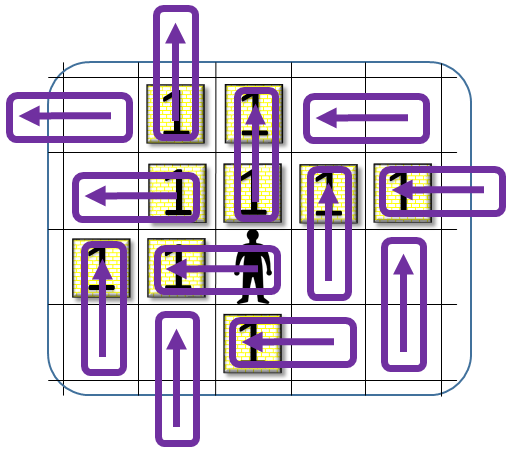## April 2017 solution

The answer is that no such minimal n exists. For every value of n and any initial configuration of 2n boxes, it is always possible to win the game. We will show an explicit strategy for doing so.

Before looking at the question of how to do this, consider the following, simpler game. This new game will begin with an empty board, divided into two half-planes: the squares with positive y coordinates, and everything else.

In our simpler game, the worker is free to move as usual, including between the two half-planes, but when it moves from the negative side of the board to the positive side, it pushes with it a new box, and all boxes must always remain in the positive half-plane. The worker's task is to compact these boxes as much as possible (which is to say, to a number of boxes that equals the Hamming weight of the binary representation of the sum of their values) before fetching the next box.

Clearly, this simpler game has easy solutions. For example, the worker can place all boxes in positions whose x and y coordinates divide by 3. This arrangement allows the worker to move any box to any other box's position without interfering with any other box. So, in particular, the worker can implement a "full adder" on the boxes, making sure that no two boxes on the board have the same value by merging any two that do have the same value before fetching a new box.

There are, in fact, far simpler solutions, closer to typical 2048 strategies (even more so if we can assume that new boxes always have either the value "1" or "2", which, as we shall see, is going to be the interesting case), but for the purpose of a mathematical proof, it's enough to know that at least one good strategy exists.

Now, let us go back to our original problem.

In any move, one can think of three participating cells: (A) where the worker started from, (B) where the worker went to, and (C) the next cell over (where a box is potentially pushed into). We usually think of a move in terms of going from (A) to (B), but obviously having any two of the three gives the full information of the move. Let us, therefore, consider moves by looking at their (B) and (C), and sketch them graphically as arrows from (B) to (C).

The reason this representation makes sense is that only the boxes at positions (B) and (C) can potentially be affected by such a move. In fact, if we think of empty cells as having value "0", then a (B)-to-(C) move is one that

1. Sets the value of (C) to the sum of the original values of (B) and (C).
2. Sets the value of (B) to zero.
3. Does not affect the value of any other position.

Now, regardless of what the original set-up of the board is and what the original position of the worker is, consider the set of moves described in the figure below.These are moves that tile the plane.

In fact, because we deal with a finite number of boxes their positions will be bounded by some finite-sized bounding box, so we only need to tile the area inside the box, and it's OK if we tile into some of the surrounding area (as is demonstrated in the image).

Going through this set of moves is possible: all the worker needs to do is to travel the board by alternating "up" and "left" moves, going back to start a new diagonal whenever it leaves the bounding box. If the original position of the worker is inside the bounding box, the first diagonal may have to be travelled twice, in order to make sure it is traversed in full, end to end.

If the worker starts at, say, position (0,0), at the end of this process, it will have emptied all positions whose x+y are 0 or 1 modulo 4, leaving only "1"s and "2"s (as well as empty places) on diagonals that are 2 or 3 modulo 4.

However, the new arrangement makes it easy for the worker to pick off whichever boxes are now closest to the edge of the bounding box and move them to be rearranged as compacted somewhere far from the bounding box, such as in a disjoint half-plane. The strategy from the easier version of the game is all that is needed at this point to complete the solution.

Q.E.D.

Readers will note that the worker's strategy is relatively "blind": the worker only needs to know its own current position and the limits of the bounding box. It does not need to know where the boxes are, or even if at any step it did any pushing.

Readers looking for an extra challenge may consider the problem of constructing a fully oblivious strategy, i.e. one that does not even require knowing where the bounding box is.

An even more extreme version (and one which I haven't analysed myself) is one where the worker does not even know where it is at each step, i.e., it is not even aware if a move attempt was successful or not.